# Physics - Classical Mechanics - Energy in Simple Harmonic Motion

in STEMGeeks5 months ago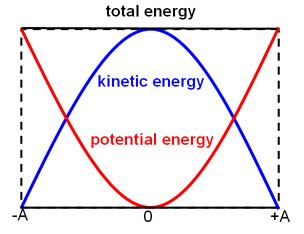[Image1]

## Introduction

Hey it's a me again @drifter1!

Today we continue with Physics, and more specifically the branch of "Classical Mechanics" in order to talk about Energy in Simple Harmonic Motion.

So, without further ado, let's dive straight into it!

## Forms of Energy in SHM

Let's continue from the horizontal mass-spring system model for simple harmonic motion that we described last time.

We already mentioned an equation which gives us the period (or frequency) from the mass m and k constant of the spring: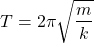But, that's not quite enough! Being a motion, we of course want to know the velocity of the mass at each point of its oscillation, as well as its position as a function of time.

Let's try approaching this problem using conservation of energy...

### Potential Energy

The first form of energy is the elastic potential energy stored in the spring when its deformed. This energy is known and given by: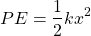Its important to note that such energy is considered to be a form of conservative energy!

### Kinetic Energy

The second form of energy is the kinetic energy of the mass due to its motion, given by: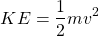which is also conservative energy.

### Total Energy

Supposing that the return force of the spring is the one and only horizontal force applied on the system, and that the mass of the spring is negligible, the total energy of the system is thus conserved and given by: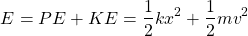### Maximum Energy

The total energy of the system depends on the amplitude A of its motion. Of course, when x = ±A the potential energy is max, and the velocity is zero (kinetic energy is zero), which yields: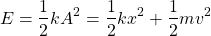### Maximum Velocity

Solving the previous equation for the velocity, now allows us to calculate the velocity, and taking it a step further the maximum velocity.

The velocity is given by: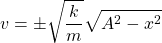For x = 0 this equation gives us the maximum velocity: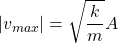## Simple Example

Let's consider a horizontal mass-spring system with a mass of m = 0.5 Kg and spring constant of k = 300 N/m. This system follows a SHM with amplitude A = 0.05 m. Calculate the following:

• Maximum energy of the system
• Maximum velocity
• Velocity at x = A / 2
• Maximum acceleration

The k constant and amplitude A are known quantities, and thus the first calculation is a simple substitution: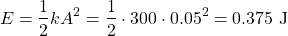Similarly, the maximum velocity is also a simple substitution: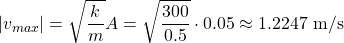For x = A / 2 we have to use the other equation, which gives us: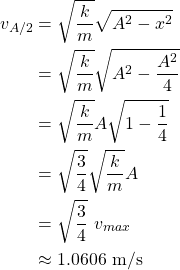For the maximum acceleration we will use the equation from the previous post and substitute x with A, because the maximum return force and in turn acceleration is at the point of most deformation: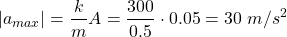## Previous articles of the series

### Periodic Motion

• Periodic Motion Fundamentals -> Fundamentals (Period, Frequency, Angular Frequency, Return Force, Acceleration, Velocity, Amplitude), Simple Harmonic Motion, Example

## Final words | Next up

And this is actually it for today's post!

Next time we will get into the mathematical equations that describe simple harmonic motion...

See ya!Keep on drifting!

Posted with STEMGeeks